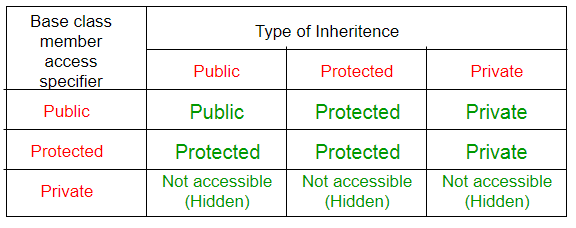# 16.1.1: Inheritance - Modes of Inheritance

$$\newcommand{\vecs}{\overset { \rightharpoonup} {\mathbf{#1}} }$$ $$\newcommand{\vecd}{\overset{-\!-\!\rightharpoonup}{\vphantom{a}\smash {#1}}}$$$$\newcommand{\id}{\mathrm{id}}$$ $$\newcommand{\Span}{\mathrm{span}}$$ $$\newcommand{\kernel}{\mathrm{null}\,}$$ $$\newcommand{\range}{\mathrm{range}\,}$$ $$\newcommand{\RealPart}{\mathrm{Re}}$$ $$\newcommand{\ImaginaryPart}{\mathrm{Im}}$$ $$\newcommand{\Argument}{\mathrm{Arg}}$$ $$\newcommand{\norm}{\| #1 \|}$$ $$\newcommand{\inner}{\langle #1, #2 \rangle}$$ $$\newcommand{\Span}{\mathrm{span}}$$ $$\newcommand{\id}{\mathrm{id}}$$ $$\newcommand{\Span}{\mathrm{span}}$$ $$\newcommand{\kernel}{\mathrm{null}\,}$$ $$\newcommand{\range}{\mathrm{range}\,}$$ $$\newcommand{\RealPart}{\mathrm{Re}}$$ $$\newcommand{\ImaginaryPart}{\mathrm{Im}}$$ $$\newcommand{\Argument}{\mathrm{Arg}}$$ $$\newcommand{\norm}{\| #1 \|}$$ $$\newcommand{\inner}{\langle #1, #2 \rangle}$$ $$\newcommand{\Span}{\mathrm{span}}$$$$\newcommand{\AA}{\unicode[.8,0]{x212B}}$$

Modes of Inheritance

1. Public mode: If we derive a sub class from a public base class. Then the public member of the base class will become public in the derived class and protected members of the base class will become protected in derived class.
2. Protected mode: If we derive a sub class from a Protected base class. Then both public member and protected members of the base class will become protected in derived class.
3. Private mode: If we derive a sub class from a Private base class. Then both public member and protected members of the base class will become Private in derived class.

Note : The private members in the base class cannot be directly accessed in the derived class, while protected members can be directly accessed. For example, Classes B, C and D all contain the variables x, y and z in below example. It is just question of access.

filter_none

// C++ Implementation to show that a derived class
// However, it does inherit a full parent object
class A
{
public:
int x;
protected:
int y;
private:
int z;
};

class B : public A
{
// x is public
// y is protected
// z is not accessible from B
};

class C : protected A
{
// x is protected
// y is protected
// z is not accessible from C
};

class D : private A // 'private' is default for classes
{
// x is private
// y is private
// z is not accessible from D
};



brightness_4

The below table summarizes the above three modes and shows the access specifier of the members of base class in the sub class when derived in public, protected and private modes: